Function Repository Resource:

# BitStringNullSpace

A memory efficient form of computing the null space of a matrix modulo 2

Contributed by: Daniel Lichtblau
 ResourceFunction["BitStringNullSpace"][{n1,n2,…},ncols] treats the integers ni as vectors of zeros and ones, each having ncols bits, and returns integers representing a set of generators of the null space modulo 2.

## Details and Options

The resulting integers represent bit strings that correspond to the null vectors form one would obtain using NullSpace with the option setting Modulus2 on the bit vectors that correspond to the input.
The number of columns must be made explicit since there might be leading zeros in all rows.
ResourceFunction["BitStringNullSpace"] is intended for dense linear algebra. Sparse methods might be better suited for linear algebra modulo 2 when most matrix entries are zeros.

## Examples

### Basic Examples (2)

Find the modulo-2 null space of a simple matrix:

 In:=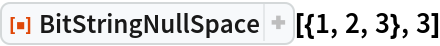Out=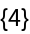Create a random matrix of bit vectors:

 In:=Out=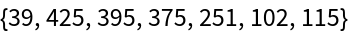Find the 0‐1 null vectors mod 2, represented as integers:

 In:=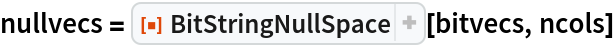Out=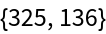### Scope (2)

Create a large array of bit strings to use as a matrix:

 In:=BitStringNullSpace is especially useful when working with matrices that might otherwise require excessive memory:

 In:=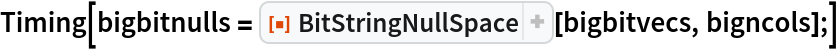Out=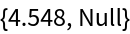### Properties and Relations (2)

Here is the matrix from which the bit vectors were created:

 In:=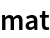Out=We see that the prior result agrees with NullSpace:

 In:=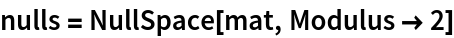Out=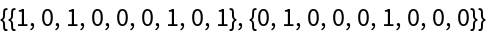In:=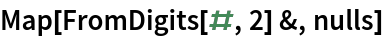Out=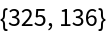In:=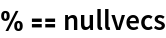Out=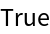Similarly, create a matrix of explicit 0‐1 vectors corresponding to the large integers:

 In:=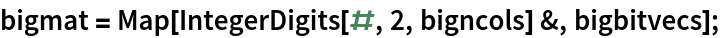NullSpace is substantially slower as compared to BitStringNullSpace:

 In:=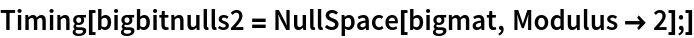Out=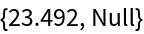Check that the results agree:

 In:=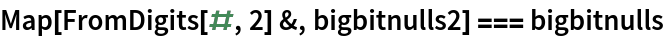Out=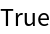## Version History

• 2.0.0 – 10 July 2019
• 1.0.0 – 03 April 2019